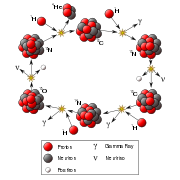# Electron capture

Nuclear physicsNuclear fission
Nuclear fusion

Electron capture (sometimes called Inverse Beta Decay) is a decay mode for isotopes that will occur when there are too many protons in the nucleus of an atom and insufficient energy to emit a positron. In such a case, a proton in the atomic nucleus captures an inner electron (that is, an electron in an inner shell) and forms a neutron and a neutrino. As a result, the number of protons within the nucleus decreases by one unit and the atom of the "parent" element is transformed into that of another ("daughter") element. The number of nucleons (protons plus neutrons) within the atomic nucleus remains unchanged.

## Contents

This process leaves the atom of the daughter element in an excited state (higher energy state). When the atom moves to the ground state (lowest energy state), it releases the extra energy in the form of an X-ray photon or an electron (called an Auger electron).

## Examples and explanation

If the energy difference between the parent atom and the daughter atom is less than 1.022 mega electronvolt (MeV), positron emission is forbidden and electron capture is the sole decay mode. Consider, for example, rubidium-83, which decays to krypton-83 solely by electron capture. The energy difference between them is about 0.9 MeV.

In this case, one of the electrons in an inner orbital, usually from the K or L electron shell, is captured by a proton in the nucleus, forming a neutron and a neutrino. Since the proton is changed to a neutron, the number of neutrons increases by one, the number of protons decreases by one, and the atomic mass number remains unchanged. By changing the number of protons, electron capture transforms the nuclide of rubidium into a nuclide of krypton. The atom moves into an excited state, with the inner shell missing an electron. When transiting to the ground state, the atom will emit an X-ray photon or Auger electron.

The general process of electron capture can be written as follows:$\mathrm{p}^+ + \mathrm{e}^- \rightarrow\mathrm{n} + {\nu}_e \,$$\mathrm{{}^{26}_{13}Al}+\mathrm{e}^- \rightarrow\mathrm{{}^{26}_{12}Mg}+{\nu}_e$$\mathrm{{}^{59}_{28}Ni}+\mathrm{e}^- \rightarrow\mathrm{{}^{59}_{27}Co}+{\nu}_e$$\mathrm{{}^{40}_{19}K}+\mathrm{e}^- \rightarrow\mathrm{{}^{40}_{18}Ar}+{\nu}_e$